gemseo / core

# dataset module¶

A generic dataset to store data in memory.

The concept of dataset is a key element for machine learning, post-processing, data analysis, …

A Dataset uses its attribute Dataset.data to store $$N$$ series of data representing the values of $$p$$ multidimensional features belonging to different groups of features.

This attribute Dataset.data is a dictionary of 2D numpy arrays, whose rows are the samples, a.k.a. series, realizations or entries, and columns are the variables, a.k.a. parameters or features. The keys of this dictionary are either the names of the groups of variables or the names of the variables. Thus, a Dataset is not only defined by the raw data stored but also by the names, the sizes and the groups of the different variables.

A Dataset can be set either from a file (Dataset.set_from_file()) or from a numpy arrays (Dataset.set_from_array()), and can be enriched from a group of variables (Dataset.add_group()) or from a single variable (Dataset.add_variable()).

An AbstractFullCache or an OptimizationProblem can also be exported to a Dataset using AbstractFullCache.export_to_dataset() and OptimizationProblem.export_to_dataset() respectively.

From a Dataset, we can easily access its length and data, either as 2D array or as dictionaries indexed by the variables names. We can get either the whole data, or the data associated to a group or the data associated to a list of variables. It is also possible to export the Dataset to an AbstractFullCache or a pandas DataFrame.

class gemseo.core.dataset.ColumnName(group, variable, component)

Bases: tuple

Create new instance of ColumnName(group, variable, component)

count(value, /)

Return number of occurrences of value.

index(value, start=0, stop=9223372036854775807, /)

Return first index of value.

Raises ValueError if the value is not present.

component

Alias for field number 2

group

Alias for field number 0

variable

Alias for field number 1

class gemseo.core.dataset.Dataset(name=None, by_group=True)[source]

Bases: object

A generic class to store data.

Parameters:
• name (str) – The name of the dataset. If None, use the name of the class.

• by_group (bool) –

If True, store the data by group. Otherwise, store them by variables.

By default it is set to True.

add_group(group, data, variables=None, sizes=None, pattern=None, cache_as_input=True)[source]

Add data related to a group.

Parameters:
• group (str) – The name of the group of data to be added.

• data (ndarray) – The data to be added.

• variables (list[str] | None) – The names of the variables. If None, use default names based on a pattern.

• sizes (dict[str, int] | None) – The sizes of the variables. If None, assume that all the variables have a size equal to 1.

• pattern (str | None) – The name of the variable to be used as a pattern when variables is None. If None, use the Dataset.DEFAULT_NAMES for this group if it exists. Otherwise, use the group name.

• cache_as_input (bool) –

If True, cache these data as inputs when the cache is exported to a cache.

By default it is set to True.

Return type:

None

Add data related to a variable.

Parameters:
• name (str) – The name of the variable to be stored.

• data (ndarray) – The data to be stored.

• group (str) –

The name of the group related to this variable.

By default it is set to “parameters”.

• cache_as_input (bool) –

If True, cache these data as inputs when the cache is exported to a cache.

By default it is set to True.

Return type:

None

compare(value_1, logical_operator, value_2, component_1=0, component_2=0)[source]

Compare either a variable and a value or a variable and another variable.

Parameters:
• value_1 (str | float) – The first value, either a variable name or a numeric value.

• logical_operator (str) – The logical operator, either “==”, “<”, “<=”, “>” or “>=”.

• value_2 (str | float) – The second value, either a variable name or a numeric value.

• component_1 (int) –

If value_1 is a variable name, component_1 corresponds to its component used in the comparison.

By default it is set to 0.

• component_2 (int) –

If value_2 is a variable name, component_2 corresponds to its component used in the comparison.

By default it is set to 0.

Returns:

Whether the comparison is valid for the different entries.

Return type:

ndarray

export_to_cache(inputs=None, outputs=None, cache_type='MemoryFullCache', cache_hdf_file=None, cache_hdf_node_name=None, **options)[source]

Export the dataset to a cache.

Parameters:
• inputs (Iterable[str] | None) – The names of the inputs to cache. If None, use all inputs.

• outputs (Iterable[str] | None) – The names of the outputs to cache. If None, use all outputs.

• cache_type (str) –

The type of cache to use.

By default it is set to “MemoryFullCache”.

• cache_hdf_file (str | None) – The name of the HDF file to store the data. Required if the type of the cache is ‘HDF5Cache’.

• cache_hdf_node_name (str | None) – The name of the HDF node to store the discipline. If None, use the name of the dataset.

Returns:

A cache containing the dataset.

Return type:

AbstractCache

export_to_dataframe(copy=True, variable_names=None, sort_names=True)[source]

Export the dataset, or a part of it, to a pandas DataFrame.

Parameters:
• copy (bool) –

If True, copy data. Otherwise, use reference.

By default it is set to True.

• variable_names (Sequence[str] | None) – The variable names to export. If None, export all the variables.

• sort_names (bool) –

If True, sort the columns by group and name. If False, sort only by group.

By default it is set to True.

Returns:

A pandas DataFrame containing the dataset, or a part of it.

Return type:

DataFrame

static find(comparison)[source]

Find the entries for which a comparison is satisfied.

This search uses a boolean 1D array whose length is equal to the length of the dataset.

Parameters:

comparison (ndarray) – A boolean vector whose length is equal to the number of samples.

Returns:

The indices of the entries for which the comparison is satisfied.

Return type:

list[int]

get_all_data(by_group=True, as_dict=False)[source]

Get all the data stored in the dataset.

The data can be returned either as a dictionary indexed by the names of the variables, or as an array concatenating them, accompanied by the names and sizes of the variables.

The data can also be classified by groups of variables.

Parameters:
• by_group

If True, sort the data by group.

By default it is set to True.

• as_dict

If True, return the data as a dictionary.

By default it is set to False.

Returns:

All the data stored in the dataset.

Return type:

Union[Dict[str, Union[Dict[str, ndarray], ndarray]], Tuple[Union[ndarray, Dict[str, ndarray]], List[str], Dict[str, int]]]

static get_available_plots()[source]

Return the available plot methods.

Return type:

list[str]

get_column_names(variables=None, as_tuple=False, start=0)[source]

Return the names of the columns of the dataset.

If dim(x)=1, its column name is ‘x’ while if dim(y)=2, its column names are either ‘x_0’ and ‘x_1’ or ColumnName(group_name, ‘x’, ‘0’) and ColumnName(group_name, ‘x’, ‘1’).

Parameters:
• variables (Sequence[str]) – The names of the variables. If None, use all the variables.

• as_tuple (bool) –

If True, return the names as named tuples. otherwise, return the names as strings.

By default it is set to False.

• start (int) –

The first index for the components of a variable. E.g. with ‘0’: ‘x_0’, ‘x_1’, …

By default it is set to 0.

Returns:

The names of the columns of the dataset.

Return type:
get_data_by_group(group, as_dict=False)[source]

Get the data for a specific group name.

Parameters:
• group (str) – The name of the group.

• as_dict (bool) –

If True, return values as dictionary.

By default it is set to False.

Returns:

The data related to the group.

Return type:

ndarray | dict[str, ndarray]

get_data_by_names(names, as_dict=True)[source]

Get the data for specific names of variables.

Parameters:
• names (str | Iterable[str]) – The names of the variables.

• as_dict (bool) –

If True, return values as dictionary.

By default it is set to True.

Returns:

The data related to the variables.

Return type:

ndarray | dict[str, ndarray]

get_group(variable_name)[source]

Get the name of the group that contains a variable.

Parameters:

variable_name (str) – The name of the variable.

Returns:

The group to which the variable belongs.

Return type:

str

get_names(group_name)[source]

Get the names of the variables of a group.

Parameters:

group_name (str) – The name of the group.

Returns:

The names of the variables of the group.

Return type:

list[str]

get_normalized_dataset(excluded_variables=None, excluded_groups=None, use_min_max=True, center=False, scale=False)[source]

Get a normalized copy of the dataset.

Parameters:
• excluded_variables (Sequence[str] | None) – The names of the variables not to be normalized. If None, normalize all the variables.

• excluded_groups (Sequence[str] | None) – The names of the groups not to be normalized. If None, normalize all the groups.

• use_min_max (bool) –

Whether to use the geometric normalization $$(x-\min(x))/(\max(x)-\min(x))$$.

By default it is set to True.

• center (bool) –

Whether to center the variables so that they have a zero mean.

By default it is set to False.

• scale (bool) –

Whether to scale the variables so that they have a unit variance.

By default it is set to False.

Returns:

A normalized dataset.

Return type:

Dataset

is_empty()[source]

Check if the dataset is empty.

Returns:

Whether the dataset is empty.

Return type:

bool

is_group(name)[source]

Check if a name is a group name.

Parameters:

name (str) – A name of a group.

Returns:

Whether the name is a group name.

Return type:

bool

is_nan()[source]

Check if an entry contains NaN.

Returns:

Whether any entries are NaN or not.

Return type:

ndarray

is_variable(name)[source]

Check if a name is a variable name.

Parameters:

name (str) – A name of a variable.

Returns:

Whether the name is a variable name.

Return type:

bool

n_variables_by_group(group)[source]

The number of variables for a group.

Parameters:

group (str) – The name of a group.

Returns:

The group dimension.

Return type:

int

plot(name, show=True, save=False, file_path=None, directory_path=None, file_name=None, file_format=None, properties=None, **options)[source]

Plot the dataset from a DatasetPlot.

Parameters:
• name (str) – The name of the post-processing, which is the name of a class inheriting from DatasetPlot.

• show (bool) –

If True, display the figure.

By default it is set to True.

• save (bool) –

If True, save the figure.

By default it is set to False.

• file_path (str | Path | None) – The path of the file to save the figures. If None, create a file path from directory_path, file_name and file_format.

• directory_path (str | Path | None) – The path of the directory to save the figures. If None, use the current working directory.

• file_name (str | None) – The name of the file to save the figures. If None, use a default one generated by the post-processing.

• file_format (str | None) – A file format, e.g. ‘png’, ‘pdf’, ‘svg’, … If None, use a default file extension.

• properties (Mapping[str, DatasetPlotPropertyType] | None) – The general properties of a DatasetPlot.

• **options – The options for the post-processing.

Return type:

DatasetPlot

remove(entries)[source]

Remove entries.

Parameters:

entries (list[int] | ndarray) – The entries to be removed, either indices or a boolean 1D array whose length is equal to the length of the dataset and elements to delete are coded True.

Return type:

None

rename_variable(name, new_name)[source]

Rename a variable.

Parameters:
• name (str) – The name of the variable.

• new_name (str) – The new name of the variable.

Return type:

None

set_from_array(data, variables=None, sizes=None, groups=None, default_name=None)[source]

Set the dataset from an array.

Parameters:
• data (ndarray) – The data to be stored.

• variables (list[str] | None) – The names of the variables. If None, use one default name per column of the array based on the pattern ‘default_name’.

• sizes (dict[str, int] | None) – The sizes of the variables. If None, assume that all the variables have a size equal to 1.

• groups (dict[str, str] | None) – The groups of the variables. If None, use Dataset.DEFAULT_GROUP for all the variables.

• default_name (str | None) – The name of the variable to be used as a pattern when variables is None. If None, use the Dataset.DEFAULT_NAMES for this group if it exists. Otherwise, use the group name.

Return type:

None

set_from_file(filename, variables=None, sizes=None, groups=None, delimiter=',', header=True)[source]

Set the dataset from a file.

Parameters:
• filename (Path | str) – The name of the file containing the data.

• variables (list[str] | None) – The names of the variables. If None and header is True, read the names from the first line of the file. If None and header is False, use default names based on the patterns the Dataset.DEFAULT_NAMES associated with the different groups.

• sizes (dict[str, int] | None) – The sizes of the variables. If None, assume that all the variables have a size equal to 1.

• groups (dict[str, str] | None) – The groups of the variables. If None, use Dataset.DEFAULT_GROUP for all the variables.

• delimiter (str) –

The field delimiter.

By default it is set to “,”.

If True, read the names of the variables on the first line of the file.

By default it is set to True.

Return type:

None

Parameters:
• name (str) – The name of the metadata attribute.

• value (Any) – The value of the metadata attribute.

Return type:

None

transform_variable(name, transformation)[source]

Transform a variable.

Parameters:
• name (str) – The name of the variable, e.g. "foo".

• transformation (Callable[[ndarray], ndarray]) – The function transforming the variable, e.g. "lambda x: np.exp(x)".

Return type:

None

DEFAULT_GROUP: ClassVar[str] = 'parameters'

The default name to group the variables.

DEFAULT_NAMES: ClassVar[dict[str, str]] = {'design_parameters': 'dp', 'functions': 'func', 'inputs': 'in', 'outputs': 'out', 'parameters': 'x'}

The default variable names for the different groups.

DESIGN_GROUP: ClassVar[str] = 'design_parameters'

The group name for the design variables of an OptimizationProblem.

FUNCTION_GROUP: ClassVar[str] = 'functions'

The group name for the functions of an OptimizationProblem.

The group name for the gradients.

HDF5_CACHE: ClassVar[str] = 'HDF5Cache'

The name of the HDF5Cache.

INPUT_GROUP: ClassVar[str] = 'inputs'

The group name for the input variables.

MEMORY_FULL_CACHE: ClassVar[str] = 'MemoryFullCache'

The name of the MemoryFullCache.

OUTPUT_GROUP: ClassVar[str] = 'outputs'

The group name for the output variables.

PARAMETER_GROUP: ClassVar[str] = 'parameters'

The group name for the parameters.

property columns_names: list[str | ColumnName]

The names of the columns of the dataset.

data: dict[str, numpy.ndarray]

The data stored by variable names or group names.

The values are NumPy arrays whose columns are features and rows are observations.

dimension: dict[str, int]

The dimensions of the groups of variables.

property groups: list[str]

The sorted names of the groups of variables.

length: int

The length of the dataset.

The metadata used to store any kind of information that are not variables,

E.g. the mesh associated with a multi-dimensional variable.

property n_samples: int

The number of samples.

property n_variables: int

The number of variables.

name: str

The name of the dataset.

property row_names: list[str]

The names of the rows.

sizes: dict[str, int]

The sizes of the variables.

strings_encoding: dict[str, dict[int, int]]

The encoding structure mapping the values of the string variables with integers.

The keys are the names of the variables and the values are dictionaries whose keys are the components of the variables and the values are the integer values.

property variables: list[str]

The sorted names of the variables.

## Examples using Dataset¶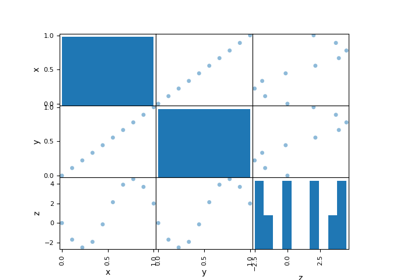Diagonal design of experiments

Diagonal design of experiments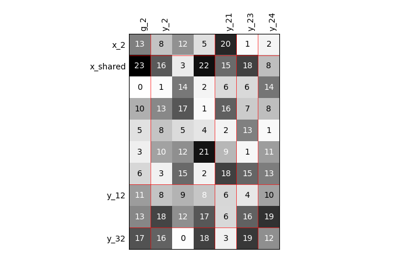Scalable diagonal discipline

Scalable diagonal disciplineCreate a surrogate discipline

Create a surrogate disciplinePlug a surrogate discipline in a Scenario

Plug a surrogate discipline in a ScenarioCreate an MDO Scenario

Create an MDO Scenario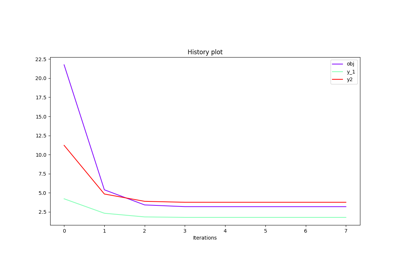Store observables

Store observables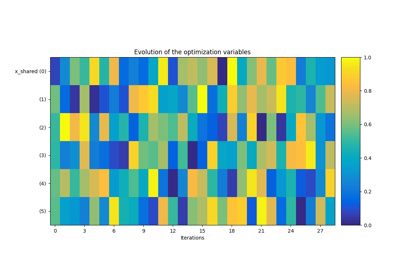BiLevel-based DOE on the Sobieski SSBJ test case

BiLevel-based DOE on the Sobieski SSBJ test case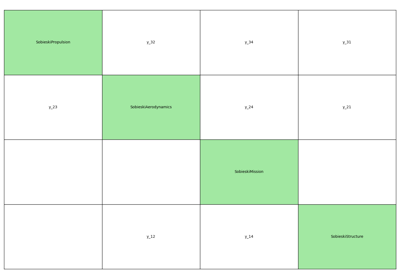MDF-based DOE on the Sobieski SSBJ test case

MDF-based DOE on the Sobieski SSBJ test caseDataset from a cache

Dataset from a cacheDataset from a numpy array

Dataset from a numpy arrayDataset from an optimization problem

Dataset from an optimization problem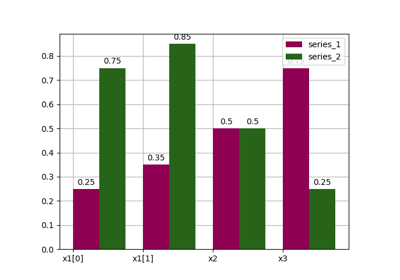Bars

Bars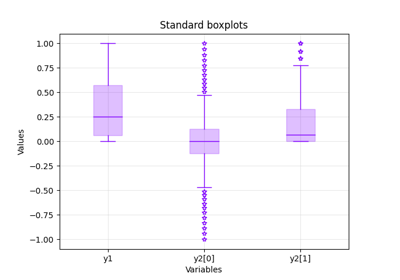Boxplot

Boxplot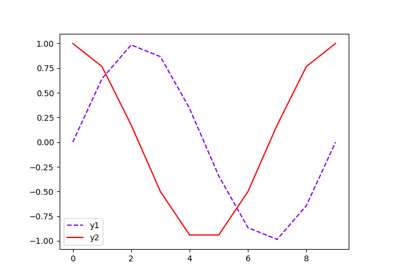Lines

Lines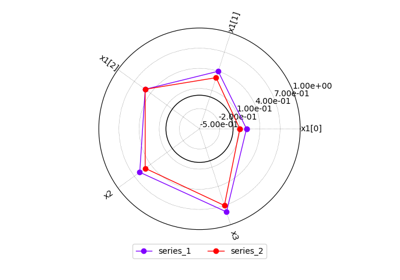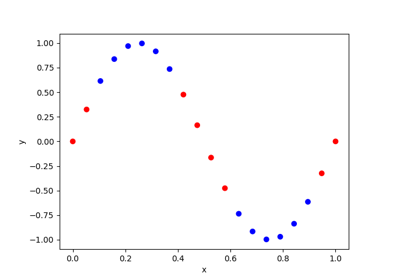Scatter

Scatter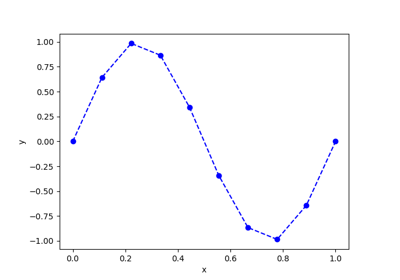YvsX

YvsX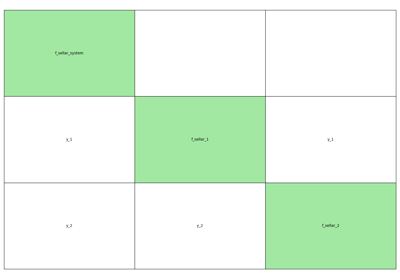GEMSEO in 10 minutes

GEMSEO in 10 minutes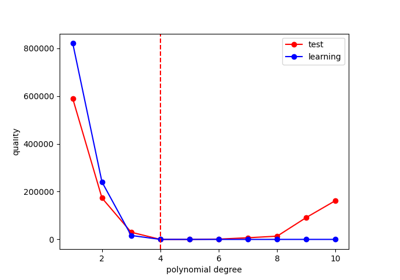Calibration of a polynomial regression

Calibration of a polynomial regression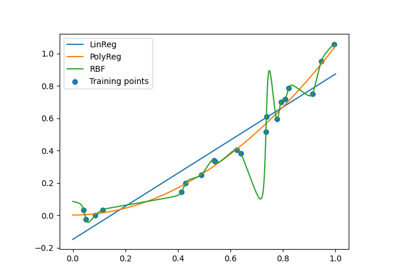Machine learning algorithm selection example

Machine learning algorithm selection example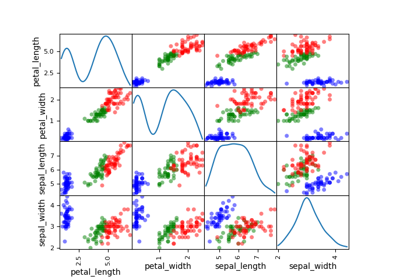Gaussian Mixtures

Gaussian Mixtures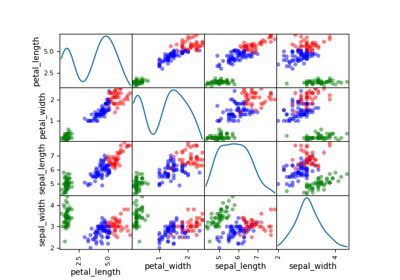K-means

K-means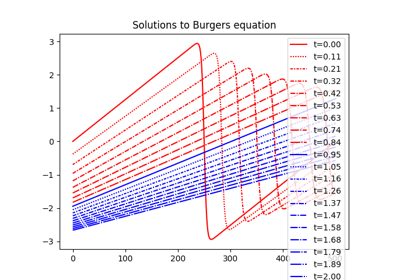KL-SVD on Burgers equation

KL-SVD on Burgers equationPCA on Burgers equation

PCA on Burgers equation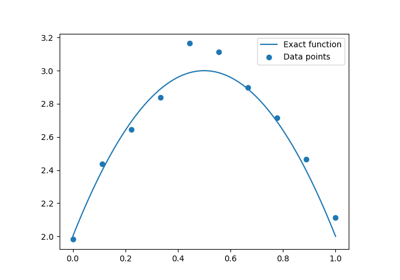MSE example - test-train split

MSE example - test-train split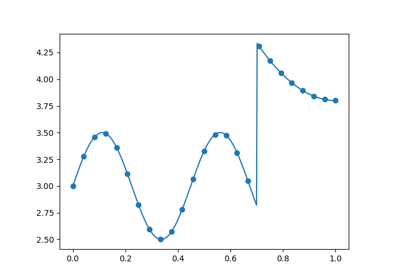Quality measure for surrogate model comparison

Quality measure for surrogate model comparisonAPI

APIGP regression

GP regressionLinear regression

Linear regressionPCE regression

PCE regressionPolynomial regression

Polynomial regressionRBF regression

RBF regressionRandom forest regression

Random forest regressionUse a design of experiments from a file

Use a design of experiments from a fileUse a design of experiments from an array

Use a design of experiments from an array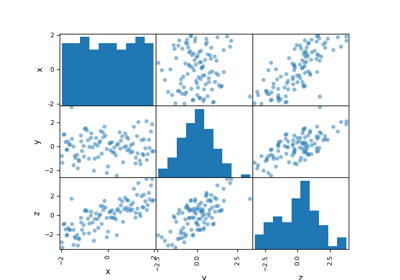Parameter space

Parameter spaceParameter space

Parameter spaceEmpirical estimation of statistics

Empirical estimation of statistics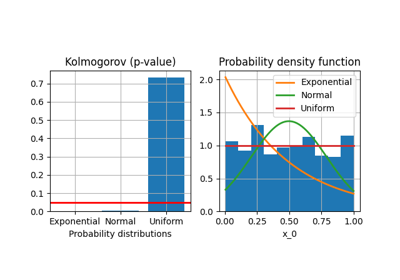Parametric estimation of statistics

Parametric estimation of statistics